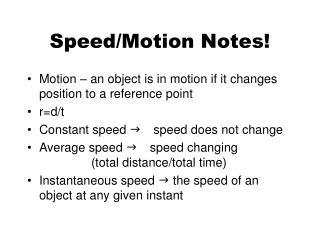DownloadDownload PresentationSpeed/Motion Notes!

# Speed/Motion Notes!

Télécharger la présentation## Speed/Motion Notes!

- - - - - - - - - - - - - - - - - - - - - - - - - - - E N D - - - - - - - - - - - - - - - - - - - - - - - - - - -
##### Presentation Transcript

1. Speed/Motion Notes! • Motion – an object is in motion if it changes position to a reference point • r=d/t • Constant speed g speed does not change • Average speed g speed changing (total distance/total time) • Instantaneous speed g the speed of an object at any given instant

2. What is the average speed of this bowling ball? S=d/t S=19m/7.67sec S=2.48m/sec

3. Slope and Speed Which trial has the greatest slope? Which trial travels the farthest in the 10 seconds? Which trial has the fastest speed? Slope = y/x = distance/time = Speed  Is short for “change in” SLOPE = SPEED!!!!!!

4. Distance= speed*time A lion runs at 50mph for .5 hr. How far does he travel? d=r*t d=50 mi/hr*.5hr d=25 mi Time= distance/speed An elephant runs at 25mph for 50 mi. How long does it take to get where he is going? t=d/r t=50mi/ 25mi/hr t=2 hr

5. A hyena travels for 30 mi. for .75 hr. How fast is the hyena traveling? r=d/t r= 30mi./.75hr r= 40 mi./hr

6. Velocity • Velocity g speed and direction! o • Boat a 6 mph east • River c 4 mph east • Velocity=6 mph east+ 4mph east • V= 10 mph east • River b 4mph west • Velocity=6mph east– 4mph west • V= 2mph east

7. Ferris Wheel The speed is constant but it is always changing velocity because it is always changing direction! http://www.ies.co.jp/math/java/trig/kanran/kanran.htmlferris wheel

8. Acceleration The rate at which velocity changes. This can be an increase in velocity (acceleration) This can be a decrease in velocity (deceleration or negative acceleration) A=(final velocity-initial velocity)/time A=(Vf-Vi)/t

9. What is the acceleration of a car that goes from 0 to 60mi/hr in 10 sec.? A=(vf-vi)/t A= (60mi/hr)/10 sec. A=6 mi/hr/sec. What is the acceleration of a car that slows down from 70mi/hr to 25 mi/hr in 15 sec.? A=(vf-vi)/t A=(25mi/hr-70mi/hr)/15 sec. A=-45mi/hr/15sec. A=-3mi/hr/sec

10. A roller coaster is constantly accelerating because the velocity is always changing! What is the acceleration of a roller coaster that starts with a velocity of 4 m/s and accelerates to 22m/s in 3 sec.? A=(22m/s-4m/s)/3s A=18m/s//3s A=6m/s/s or 6m/s2

11. Gravity! Gravity: a force that pulls objects together. Freefall: a falling object with no other force but gravity acting upon it. Acceleration due to gravity is 9.8m/s/s

12. The person jumps off the plane. What is his speed at 1 sec? 2 sec? 3 sec?

13. Terminal Velocity! The maximum velocity a falling object can achieve. The air resistance equals the force of gravity so the object does not accelerate any more. http://hypertextbook.com/facts/JianHuang.shtml